Figures for "Wild knots"

Cameron Browne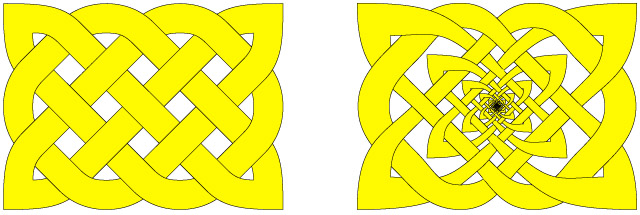Figure 1. A simple 3:4 Celtic knot (left) and a wild knot based on the same design (right).Figure 2. The generator set showing cord attenuation rules a, b and c (left) and the outer frame (right).Figure 3. The wild knot at generation one (left) and its terminating plug (right).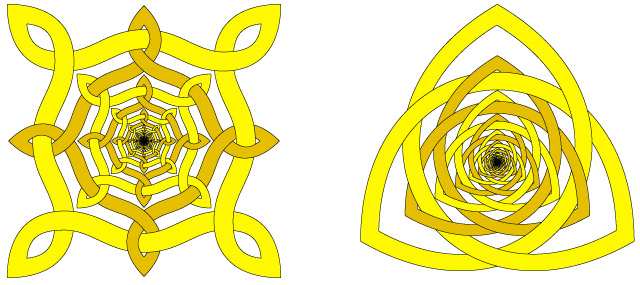Figure 4. Tame fractal knotwork designs.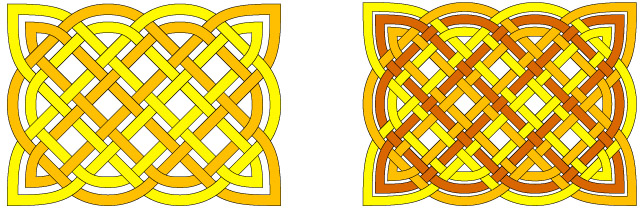Figure 5. The basic 3:4 plait as a 2-interlaced design (left) and a 3-interlaced design (right).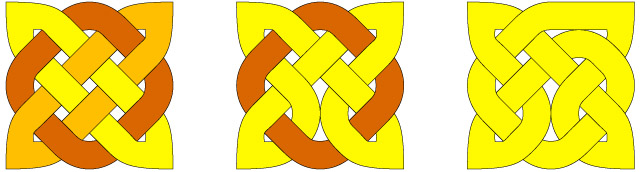Figure 6. Break operations reduce three cords (left) to two cords (middle) to one cord (right).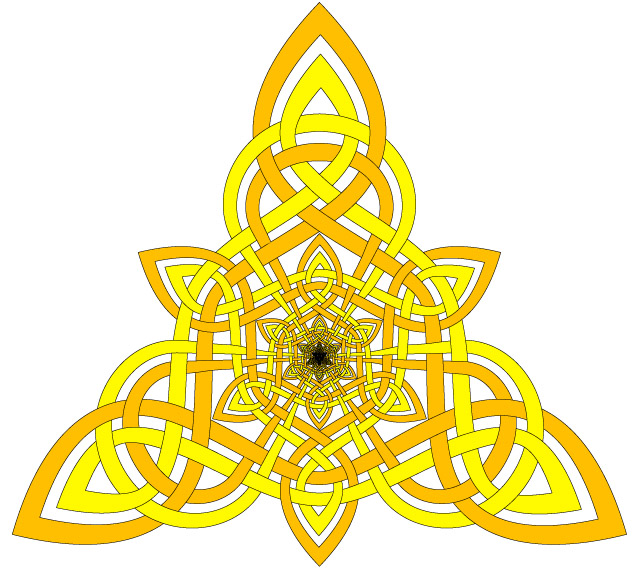Figure 7. A hybrid wild fractal 2-interlaced knotwork design.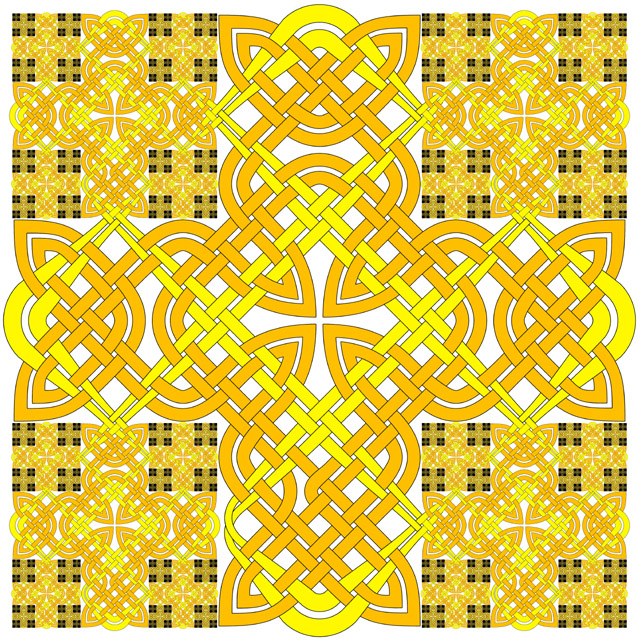Figure 8. A more complex hybrid wild fractal 2-interlaced knotwork design.